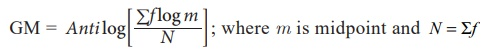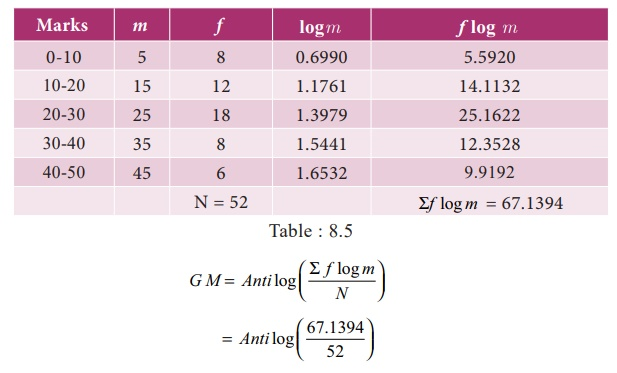Home | | Business Maths 11th std | Geometric mean

# Geometric mean

Geometric mean is defined as the nth root of the product of n observations or values.

Geometric mean

Geometric mean is defined as the nth root of the product of n observations or values.

If there are two observations, we take the square root; if there are three observations we have to take the cube root and so onwhere X 1 , X2 , X 3 ,...., Xn refer to the various items of the series which are all greater than zero and n refers number of observations.

Thus the geometric mean of 3 values 2,3,4 would beWhen the number of items is three or more the task of multiplying the numbers and of extracting the root becomes excessively difficult. To simplify calculations, logarithms are used. Geometric mean is calculated as follows:(i) In discrete observation(ii)  In Continuous observationExample 8.5

Daily income (in Rs) of ten families of a particular place is given below. Find out GM

85, 70, 15, 75, 500, 8, 45, 250, 40, 36

Solution:Anti log(1.7637)

GM = 58.03

Example 8.6

Calculate the geometric mean of the data given below giving the number of families and the income per head of different classes of people in a village of Kancheepuram District.Solution:

Calculation of Geometric MeanAnti log (1.8687)

GM = 73.95

Example 8.7

Compute the Geometric mean from the data given belowSolution:Anti log (1.2911)

GM = 19.55

Specific uses of Geometric mean

The most useful application of geometric mean is to average the rate of changes. For example, from 2006 to 2008 prices increased by 5%, 10% and 18% respectively.

The average annual increase is not 11% (5 + 10 + 18) / 3 = 11 as given by the arithmetic average but 10.9% as obtained by the geometric mean. This average is also useful in measuring the growth of population, because population increases in geometric progression.

Example 8.8

Compared to the previous year the overhead expenses went up by 32% in 1995, they increased by 40% in the next year and by 50% in the following year. Calculate the average rate of increase in overhead expenses over the three years.

Solution:

In averaging ratios and percentages, geometric mean is more appropriate. Let us consider X represents Expenses at the end of the year.Anti log ( 2.1476)

GM = 140.5

Average rate of increase in overhead expenses

140.5 â€“ 100 = 40.5 %

Tags : Measures of central tendency , 11th Business Mathematics and Statistics(EMS) : Chapter 8 : Descriptive statistics and probability
Study Material, Lecturing Notes, Assignment, Reference, Wiki description explanation, brief detail
11th Business Mathematics and Statistics(EMS) : Chapter 8 : Descriptive statistics and probability : Geometric mean | Measures of central tendency